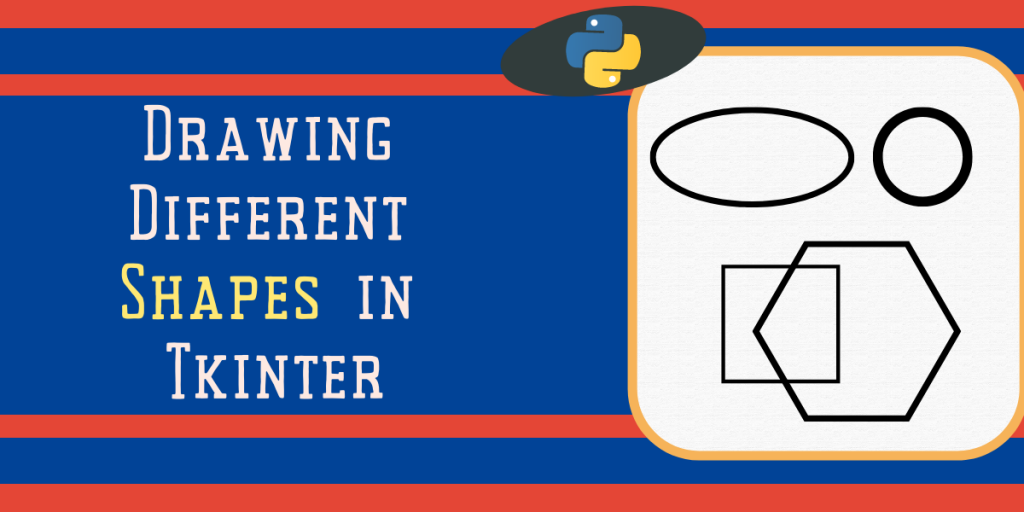# How to Draw Different Shapes Using TkinterIn this tutorial, we’ll learn how to draw different shapes in Tkinter such as square, circle, rectangle, oval, arc, etc. In Tkinter, the canvas class is used to draw different shapes in our application window. Functions to draw shapes are defined in canvas class so we need to define the canvas class first.

Follow the below step-by-step tutorial to draw different shapes in Python GUI using Tkinter.

Also read: Drawing Lines using Tkinter – Basics for Beginners

## Set up Tkinter

To install the Tkinter module run the below command in the terminal.

```pip install tk
```

To import the installed module, we import all the methods in the Tkinter library by using *:

```from tkinter import *
```

## Drawing Shapes in Python Tkinter Canvas

First, we need to initialize the Tkinter and canvas class. Tkinter Canvas class contains every method for creating different shapes.

```root = Tk()
canvas = Canvas()
root.mainloop()
```

After initialization of the Tkinter and canvas class, we start with the drawing of different shapes

### 1. Oval

Oval can be easily drawn using the create_oval() method. This method takes coordinates, color, outline, width, etc. as a parameter. All shapes are created inside a box whose coordinates we provide.

```create_oval(x0, y0, x1, y1)
```

In the above code, we’ve set the coordinates of the box so it makes a perfect square and inside that square, we’ll have our perfect circle.

```canvas.create_oval(10, 10, 80, 80, outline = "black", fill = "white",width = 2)
canvas.pack()
```

### 2. Circle

There is no special function for creating a circle, it is drawn by using the create_oval() function. We just need to keep the horizontal length the same as the vertical length. Mathematically, meaning the radius of the shape(circle) is equal to all sides of the boundary(diameter).

```canvas.create_oval(110,10,210,110,outline = "black",fill = "white",width = 2)
canvas.pack()
```

### 3. Square or Rectangle

By using the create_rectangle method we draw a rectangle and square shapes. Here we pass the edges/sides of our shape and hence can draw a square also, using the same method (all sides equal).

```canvas.create_rectangle(10,10,110,110,outline ="black",fill ="white",width = 2)
canvas.create_rectangle(210,10,310,210,outline ="black",fill ="white",width =2)
canvas.pack()
```Draw Square Or Rectangle In Tkinter

### 4. Polygon

We can draw as many vertices as we want. We use the create_polygon() method which takes coordinates of edges and renders them accordingly on our main window. In the below code we’ve created a list of coordinates and passed it into our create_polygon method.

```#points for the vertices in x1,y1,x2,y2, and so on

points = [150, 100, 200, 120, 240, 180, 210, 200, 150, 150, 100, 200]

canvas.create_polygon(points, outline = "blue", fill = "orange", width = 2)
canvas.pack()
```

### 5. Arc

We create arc shapes by using create_arc method.